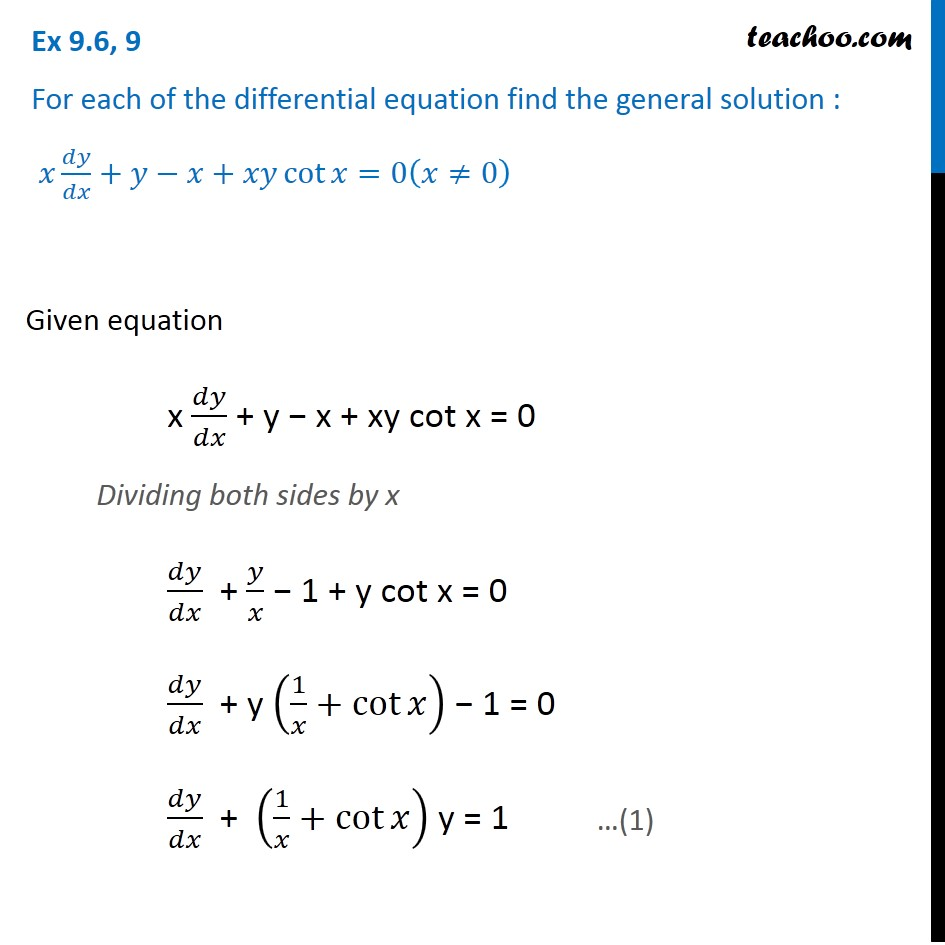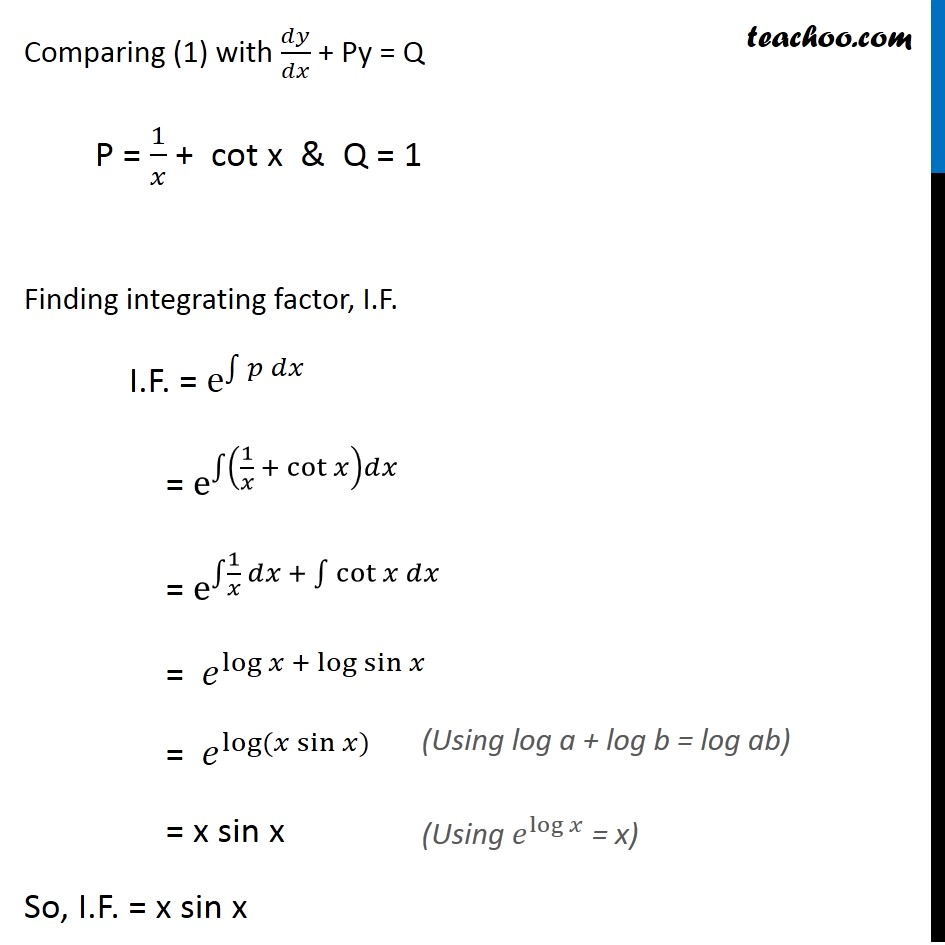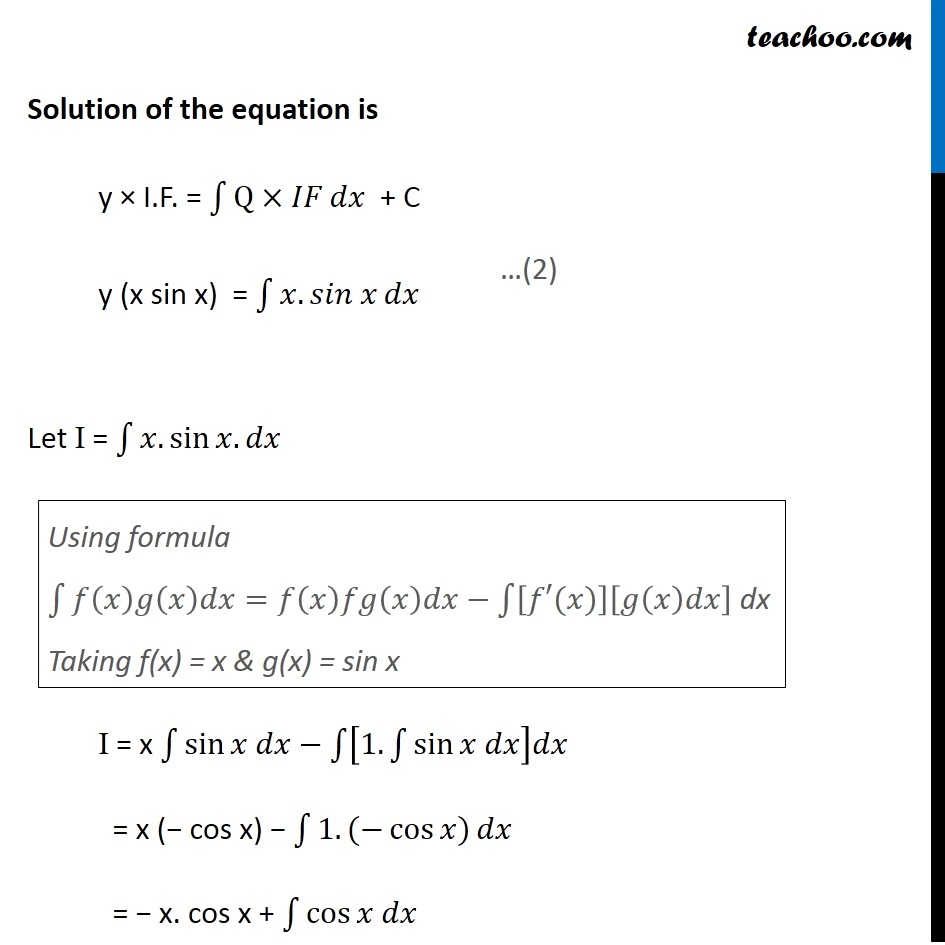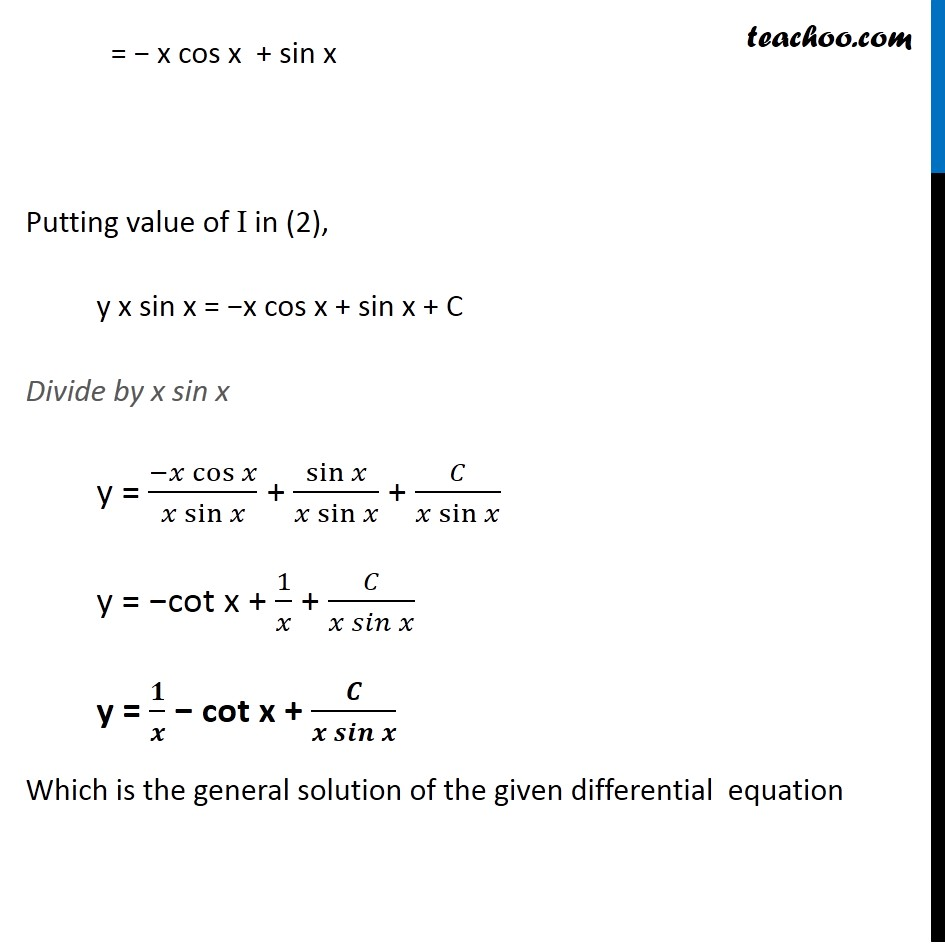Ex 9.5

Chapter 9 Class 12 Differential Equations
Serial order wiseLearn in your speed, with individual attention - Teachoo Maths 1-on-1 Class

### Transcript

Ex 9.5, 9 For each of the differential equation find the general solution : 𝑥 𝑑𝑦/𝑑𝑥+𝑦−𝑥+𝑥𝑦 cot⁡〖𝑥=0(𝑥≠0)〗 Given equation x 𝑑𝑦/𝑑𝑥 + y − x + xy cot x = 0 Dividing both sides by x 𝑑𝑦/𝑑𝑥 + 𝑦/𝑥 − 1 + y cot x = 0 𝑑𝑦/𝑑𝑥 + y (1/𝑥+cot⁡𝑥 ) − 1 = 0 𝑑𝑦/𝑑𝑥 + (1/𝑥+cot⁡𝑥 ) y = 1 …(1) Comparing (1) with 𝑑𝑦/𝑑𝑥 + Py = Q P = 1/𝑥 + cot x & Q = 1 Finding integrating factor, I.F. I.F. = e^∫1▒〖𝑝 𝑑𝑥 〗 = e^∫1▒(1/𝑥 + cot⁡𝑥 )𝑑𝑥 = e^∫1▒〖1/𝑥 𝑑𝑥 + ∫1▒〖cot⁡𝑥 𝑑𝑥〗〗 = 𝑒^(log⁡𝑥 + log⁡sin⁡𝑥 ) = 𝑒^log⁡〖(𝑥 sin⁡𝑥)〗 = x sin x So, I.F. = x sin x (Using log a + log b = log ab) (Using 𝑒^log⁡𝑥 = x) Solution of the equation is y × I.F. = ∫1▒〖Q×𝐼𝐹〗⁡𝑑𝑥 + C y (x sin x) = ∫1▒〖𝑥.〖𝑠𝑖𝑛 𝑥〗⁡𝑑𝑥 〗 Let I = ∫1▒〖𝑥.sin⁡〖𝑥.𝑑𝑥〗 〗 I = x ∫1▒sin⁡〖𝑥 𝑑𝑥−∫1▒[1.∫1▒sin⁡〖𝑥 𝑑𝑥〗 ]𝑑𝑥〗 = x (− cos x) − ∫1▒〖1.(−cos⁡〖𝑥)〗 𝑑𝑥〗 = − x. cos x + ∫1▒cos⁡〖𝑥 𝑑𝑥〗 …(2) Using formula ∫1▒〖𝑓(𝑥)𝑔(𝑥)𝑑𝑥=𝑓(𝑥)𝑓𝑔(𝑥)𝑑𝑥−∫1▒[𝑓′(𝑥)][𝑔(𝑥)𝑑𝑥] 〗 dx Taking f(x) = x & g(x) = sin x = − x cos x + sin x Putting value of I in (2), y x sin x = −x cos x + sin x + C Divide by x sin x y = (−𝑥 cos⁡𝑥)/(𝑥 sin⁡𝑥 ) + sin⁡𝑥/(𝑥 sin⁡𝑥 ) + 𝐶/(𝑥 sin⁡𝑥 ) y = −cot x + 1/𝑥 + 𝐶/(𝑥 𝑠𝑖𝑛⁡𝑥 ) y = 𝟏/𝒙 − cot x + 𝑪/(𝒙 𝒔𝒊𝒏⁡𝒙 ) Which is the general solution of the given differential equation